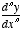nth derivative

nth Derivative

The result of taking the derivative of the derivative of the derivative etc. of a function a total of n times. Written
f (n)(x) or.

Note: f (0)(x) is the same thing as f(x).